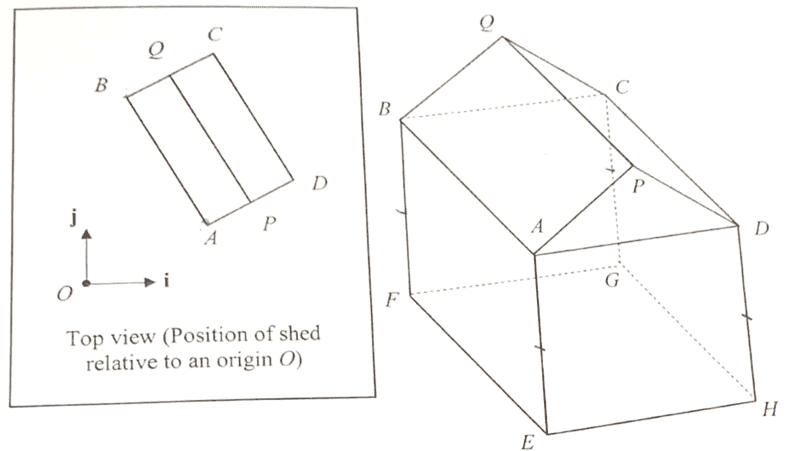# Normal vector of plane

• songoku

#### songoku

Homework Statement
The planes ABCD and EFGH are parallel to the horizontal surface represented by the xy-plane. The triangles APD and BQC are congruent isosceles triangles and the lengths of the pillars AE, BF, CG, and DH are of the same height. The equation of the plane ABQP is given by -2x-y+3z = 2 and the point P has position vector 4i + 2j + 4k.

Explain why plane CDPQ is perpendicular to 2i + j + 3k. Hence show that the equation of the plane CDPQ is 2x + y + 3z = 22
Relevant Equations
Equation of plane: r . n = cI know the normal of plane ABQP is -2i - j + 3k but I don't know how to prove that 2i + j + 3k is the normal vector of plane CDPQ

Thanks

Hi, hope you are in the fullest of your sprits!
To find the normal, you can use the vector product
If you don't know what that is, thenwhere ||A|| and ||B|| are magnitudes of vectors A and B respectively

•songoku
Hi, hope you are in the fullest of your sprits!
To find the normal, you can use the vector product
If you don't know what that is, thenwhere ||A|| and ||B|| are magnitudes of vectors A and B respectively
The formula shown is somewhat unclear, as it looks like the last factor is ##\sin (\theta n)##. In fact, the formula should be as follows:
$$\vec A \times \vec B = \left(|\vec A| |\vec B| \sin(\theta)\right) \vec n$$
where ##\vec n## is the unit vector that is perpendicular to both ##\vec A## and ##\vec B##.

•songoku
Hi, hope you are in the fullest of your sprits!
Hello @Cuckoo Beats ,•PhDeezNutz and songoku
I know the normal of plane ABQP is -2i - j + 3k but I don't know how to prove that 2i + j + 3k is the normal vector of plane CDPQ

•